# Standard Equations of Parabola

In conic sections, a parabola is the set of all points in a plane that are equidistant from a fixed line and a fixed point (not on the line) in the plane. The fixed line is called the directrix, and the fixed point (F) is called the parabola’s focus. A line through the focus and perpendicular to the directrix is called the axis of the parabola. Also, the vertex is the point of intersection of a parabola with the axis. As we know, the standard equation of the parabola is used in solving a variety of problems in maths. In this article, you will learn how to write the standard equations for parabola in different cases and how to solve questions based on these equations.

## Standard Equation for Parabola

In this section, one can learn what is the standard equation of a parabola and how to write the equations of parabola. The parabola equation is simplest if the vertex is at the origin and the axis of symmetry is along the x-axis and y-axis. The four such possible orientations of the parabola are explained in the table below:

 Equation Parabola Formulas y2 = 4ax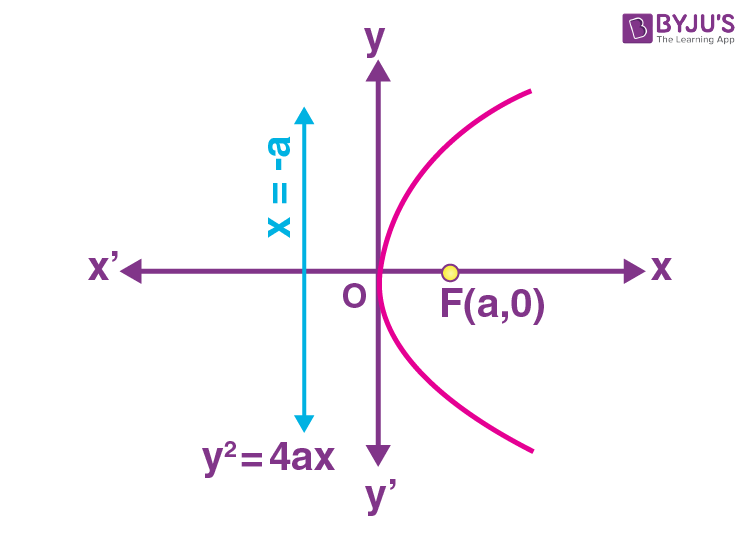Focus = (a, 0); a > 0 Directrix: x = -a (or) x + a = 0 Length of the Latus rectum = 4a Axis: Positive x-axis y2 = -4ax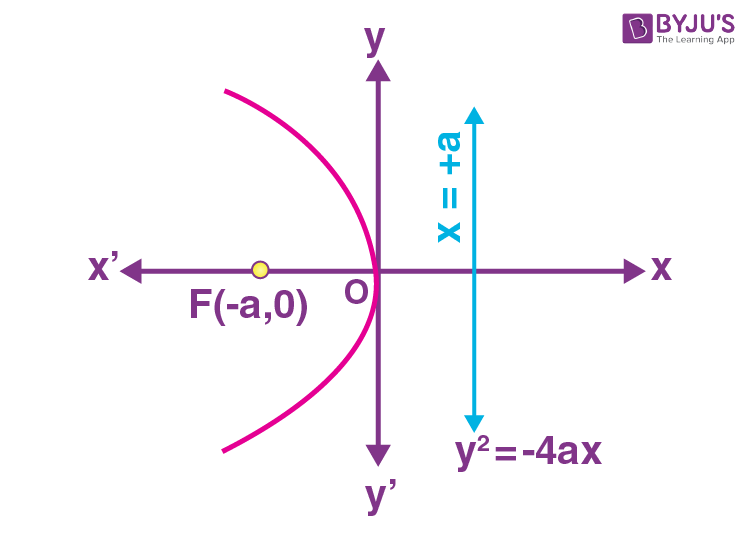Focus = (-a, 0); a < 0 Directrix: x = a (or) x – a = 0 Length of the Latus rectum = 4a Axis: Negative x-axis x2 = 4ay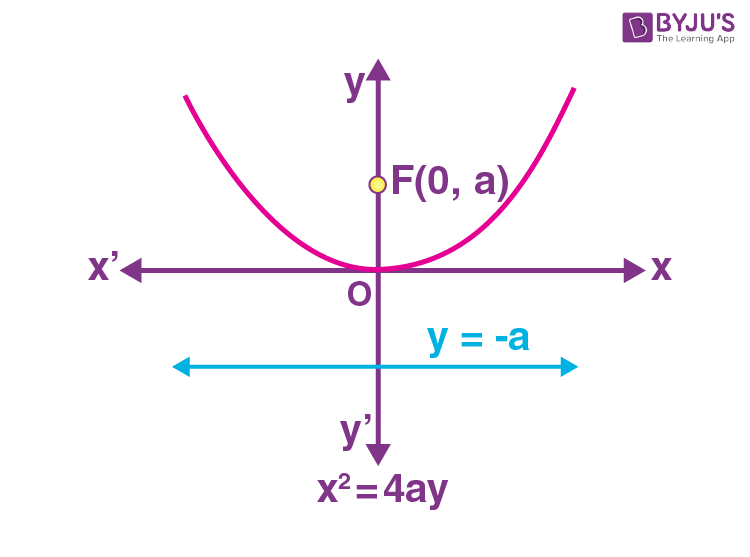Focus = (0, a); a > 0 Directrix: y = -a (or) y + a = 0 Length of the Latus rectum = 4a Axis: Positive y-axis x2 = -4ay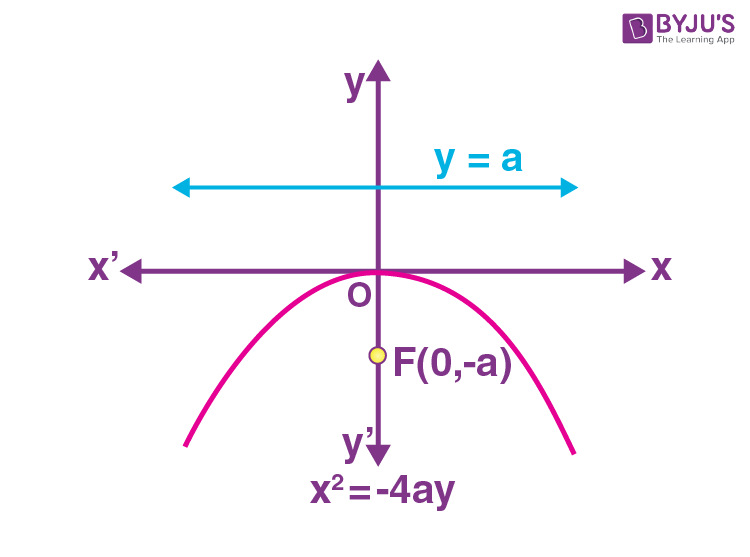Focus = (0, -a); a < 0 Directrix: y = a (or) y – a = 0 Length of the Latus rectum = 4a Axis: Negative y-axis

Thus, we can derive the equations of the parabolas as:

y2 = 4ax

y2 = -4ax

x2 = 4ay

x2 = -4ay

These four equations are called standard equations of parabolas. It is important to note that the standard equations of parabolas focus on one of the coordinate axes, the vertex at the origin. The directrix is parallel to the other coordinate axis. However, we can list the following observations from the above equations of parabolas in standard form.

• Parabola is symmetric to the axis of the parabola.
• If the equation has a y2 term, then the axis of symmetry is along the x-axis. In this case, the parabola opens to the-

(a) right if the coefficient of x is positive

(b) left if the coefficient of x is negative
• If the equation has an x2 term, then the axis of symmetry is along the y-axis. In this case, the parabola opens-

(c) upwards if the coefficient of y is positive

(d) downwards if the coefficient of y is negative

#### Watch The Below Video To Understand the Orientations of ParabolaNow, let us write the equation of parabola in standard form when the vertex is not at the origin and the axis of symmetry is parallel to the x-axis or y-axis. These are tabulated as given below:

 Equation Parabola Formulas (y – k)2 = 4a(x – h)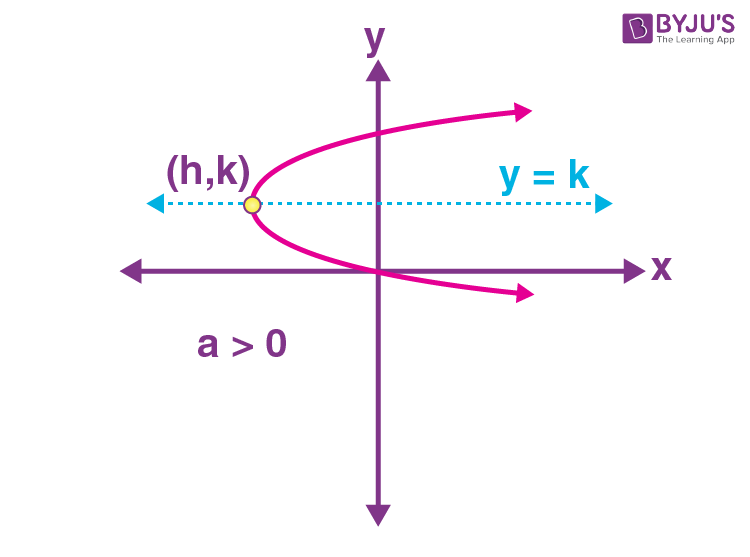Vertex (h, k) Parabola opens to the right side Focus: (h + a, k) Directrix: x = h – a Axis: y = k (y – k)2 = -4a(x – h)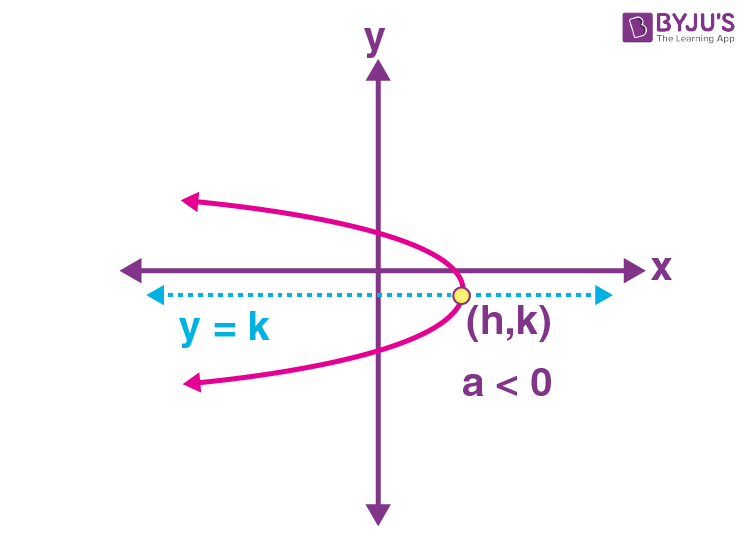Vertex (h, k) Parabola opens to the Left side Focus: (h – a, k) Directrix: x = h + a Axis: y = k (x – h)2 = 4a(y – k)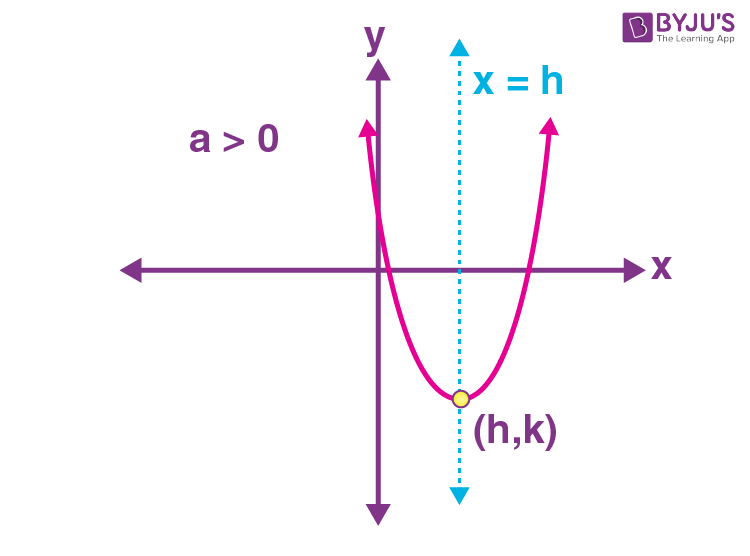Vertex: (h, k) Parabola opens to the upward Focus: (h, k + a) Directrix: y = k – a Axis: x = h (x – h)2 = -4a(y – k)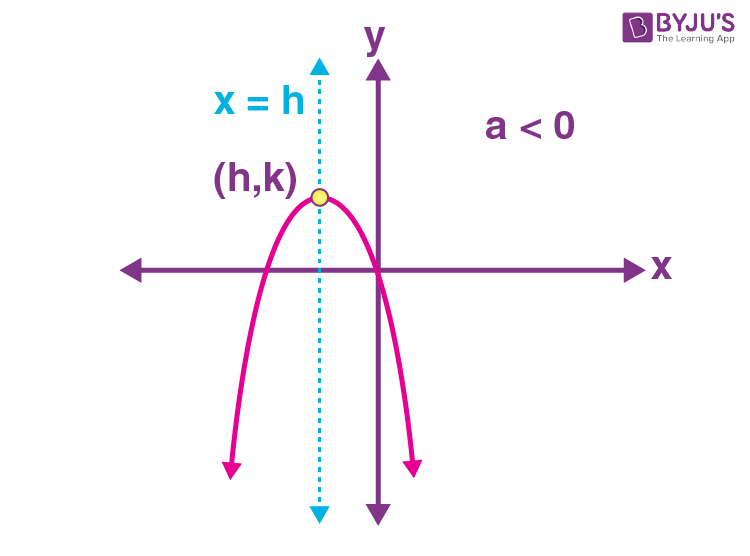Vertex: (h, k) Parabola opens to the downward Focus: (h, k – a) Directrix: y = k + a Axis: x = h

### Solved Examples

Example 1: Find the coordinates of the focus, axis, the equation of the directrix and latus rectum of the parabola y2 = 12x.

Solution:

Given equation of the parabola is: y2 = 12x

Comparing with the standard form y2 = 4ax,

4a = 12

a = 3

The coefficient of x is positive so the parabola opens

to the right.

Also, the axis of symmetry is along the positive x-axis.

Therefore,

Focus of the parabola is (a, 0) = (3, 0).

Equation of the directrix is x = -a, i.e. x = -3 or x + 3 = 0.

Length of the latus rectum = 4a = 4(3) = 12

Example 2: Find the equation of the parabola which is symmetric about the y-axis, and passes through the point (3, -4).

Solution:

Given that the parabola is symmetric about the y-axis and has its vertex at the origin.

Thus, the equation can be of the form x2 = 4ay or x2 = -4ay, where the sign depends on whether the parabola opens upwards or downwards.

Since the parabola passes through (3, -4) which lies in the fourth quadrant, it must open downwards.

So, the equation will be: x2 = -4ay

Substituting (3, -4) in the above equation,

(3)2 = -4a(-4)

9 = 16a

a = 9/16

Hence, the equation of the parabola is: x2 = -4(9/16)y

Or

4x2 = -9y

Go through the practice questions given below to get a thorough understanding of the different cases of parabolas explained above.

### Practice Problems

1. Find the equation of the parabola with focus (4, 0) and directrix x = -4.

2. Find the equation of the parabola whose vertex is (0, 0), passing through (5, 2) and symmetric with respect to y-axis.

3. Given the equation of parabola: (x – 2)2 = -8(y – 3)

State whether the parabola opens upward, downward, right or left, and also write the coordinates of the vertex, the focus, and the equation of the directrix.

4. Find the focus and directrix of the parabola whose equation is y2 – 6y + 3x + 18 = 0.